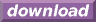#Calcute: freeware calculator

## Division (div, /)

A division is the inverse of a multiplication. Calcute implements it using either the div function or, equivalently, the / (division) operator. Given three values 'q', 'r' and 's' such that q/r = s then q = s*r.

```div(30; 6)
5

30 / 6
5
```

In a division, the value being divided is called the "divend". The value by which the dividend is divided is called the "divisor". The result of the division is called the "quotient".